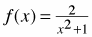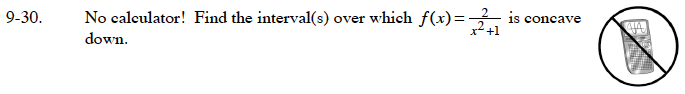### Home > CALC > Chapter 9 > Lesson 9.1.2 > Problem9-30

9-30.

No calculator! Find the interval(s) over whichis concave down. Homework Help ✎Homework Help ✎If you differentiate this function correctly, the second derivative is:

$f^{\prime\prime}(x)=\frac{4(3x^2-1)}{(x^2+1)^3}$

The graph of the function is concave down when f ″(x) < 0.